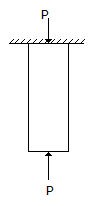# Civil Engineering - Theory of Structures

Exercise : Theory of Structures - Section 3
46.
Keeping breadth constant, depth of a cantilever of length l of uniform strength loaded with uniformly distributed load w varies from zero at the free end and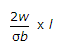at the fixed end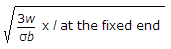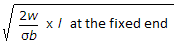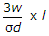at the fixed end
Explanation:
No answer description is available. Let's discuss.

47.
The force in CD of the truss shown in given figure, is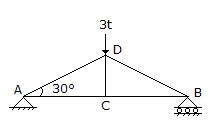3t compression
3t tension
zero
1.5t compression
1.5t tension
Explanation:
No answer description is available. Let's discuss.

48.
The deflection of a uniform circular bar of diameter d and length l, which extends by an amount e under a tensile pull W, when it carries the same load at its mid-span, is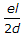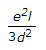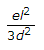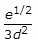Explanation:
No answer description is available. Let's discuss.

49.
The ratio of the stresses produced by a suddenly applied load and by a gradually applied load on a bar, is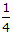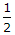1
2
3